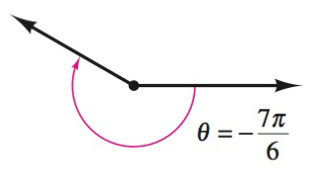Chapter 8.1, Problem 8E### Calculus: An Applied Approach (Min...

10th Edition
Ron Larson
ISBN: 9781305860919

#### Solutions

Chapter
Section### Calculus: An Applied Approach (Min...

10th Edition
Ron Larson
ISBN: 9781305860919
Textbook Problem
1 views

# Finding Conterminal Angles In Exercise 7-10, determine two conterminal angles in radian measure (one positive and one negative) for the angle.To determine

To calculate: The two coterminal angles in radian measure, in which one is positive and another is negative with the help of provided diagram,Explanation

Given Information:

The provided angle is θ=7π6 radians, which is shown below as:

Formula used:

The angle measuring θ has same initial and terminal sides as the angle measuring 360θ, such angles are coterminal angle.

Calculation:

Draw diagram for coterminal angles and provided angle for θ=7π6 radians.

From above diagram, let θ+ be the positive coterminal angle and θ be the negative coterminal angle

### Still sussing out bartleby?

Check out a sample textbook solution.

See a sample solution

#### The Solution to Your Study Problems

Bartleby provides explanations to thousands of textbook problems written by our experts, many with advanced degrees!

Get Started

#### In Exercises 13-20, sketch a set of coordinate axes and plot each point. 16. (3, 4)

Applied Calculus for the Managerial, Life, and Social Sciences: A Brief Approach

#### If 13f(x)dx=10 and 13g(x)dx=6, then 13(2f(x)3g(x))dx= a) 2 b) 4 c) 18 d) 38

Study Guide for Stewart's Single Variable Calculus: Early Transcendentals, 8th

#### What are the five steps of the scientific method?

Research Methods for the Behavioral Sciences (MindTap Course List)